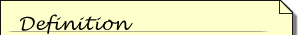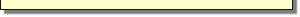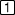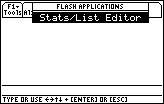Module 3 - Logistic Growth

Introduction | Lesson 1 | Lesson 2 | Lesson 3 | Self-Test

Introduction

Many traditional textbook problems begin with an equation and then produce the graph of the equation. However, in many real-world problems researchers first gather and graph data and then attempt to find an equation that fits the data. Technology facilitates this real-world approach to functions and graphs.

In this module you will learn to use the TI-89 to create a scatter plot ofLogistic functions are used to represent growth that has a limiting factor, such as food supplies, war, new diseases, etc. Logistic models are often used to model population growth or the spread of disease or rumor.logistic data and then find aAn equation that represents a set of data is called a regression equation, and it is used to estimate or predict values of a data set.regression equation that fits the data. You will use the TI-89 Statistics and List Editor, an application that is found in newer TI-89's or can be freely downloaded from TI's web site.

To find out if your calculator has the Statistics and List Editor application,

• Display the Flash Apps menu by pressingIf one of the menu items is "Stats/List Editor," you are ready to proceed with this module.If you do not have this feature, obtain the free upgrade from the TI Education web site at http://education.ti.com/educationportal/sites/US/productDetail/us_ti89ti.html. You will need a Graph Link cable and TI-Graph Link(89) software to install the upgrade from your computer to your calculator.

Lesson Index:

3.1 - Scatter plots

3.2 - Curve fitting

3.3 - Change in y

After completing this module, you should be able to do the following:

• Enter data in the TI-89 Stats/List Editor
• Make a scatter plot
• Find and graph a regression equation to fit a data set
• Calculate and graph the changes in y
• Understand and describe what the changes in y tell you about the original data

Next >